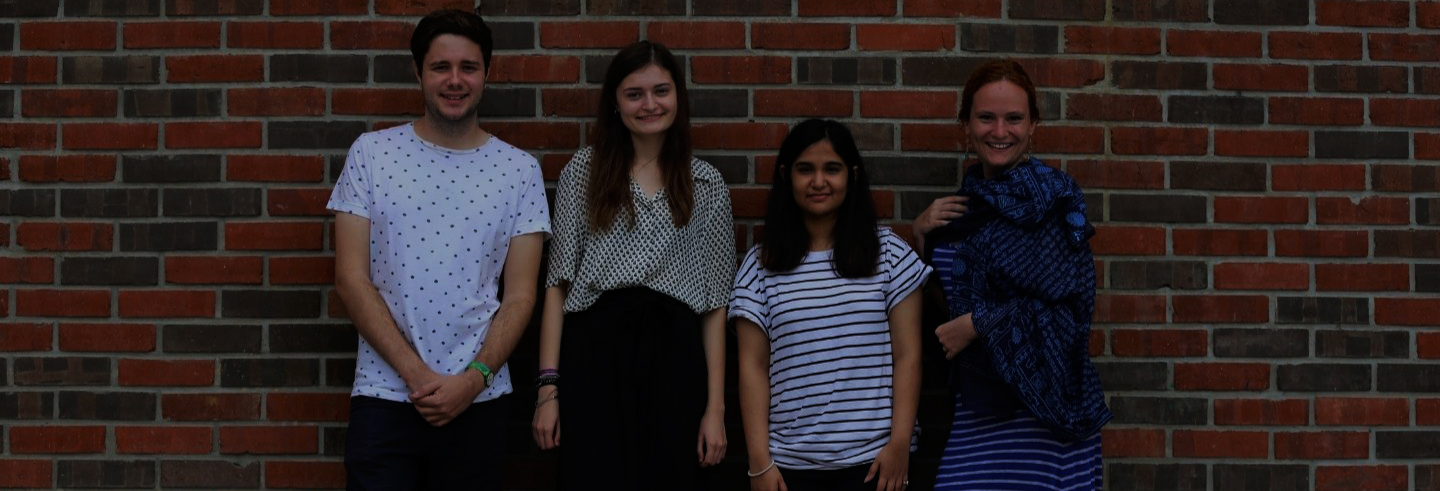Some of the most powerful and beautiful ideas occur in the field of Mathematics. The wide applicability of these ideas and their deep connection with the natural sciences have made this discipline one of the most fruitful arenas of human inquiry. Combining, as it does, the greatest creative freedom with the most stringent standards of rigor, Mathematics also happens to be the ideal training ground for learning a broad range of analytical and problem-solving skills. Ashoka University’s Mathematics major program has been designed to meet two primary goals:

1. Students should get a broad exposure to the primary areas and the central ideas of contemporary Mathematics (as well as their applications) and
2. Students should develop rigorous, analytical reasoning skills, along with problem-solving ability.

In addition to the Foundation courses that are common across disciplines, students aiming to choose Mathematics as their major should take the enabling course on Calculus as early as possible. This will be followed by 11 required and 3 elective courses in Mathematics. The required courses cover the areas of Analysis, Algebra, Linear Algebra, Probability, and Differential Equations. Elective courses vary from semester to semester depending on student and faculty interests. In addition, students have the opportunity to study some special topics in depth under the guidance of a faculty member.

At the end of the program of study, we expect students to be able to read and understand mathematical proofs; learn and apply new mathematical concepts; and, construct and communicate a correct and rigorous argument on their own. Most importantly, we expect students to be able to solve new mathematical problems on their own. Students completing this program will be well prepared to pursue Mathematics further or to take up positions that call for innovative problem solving in concert with strong analytical abilities.

Students may also opt for an inter-disciplinary BSc (Hons.) with Major in Mathematics and Computer Science. This allows them to get a wider variety of problem-solving skills as well as make them more attractive to the industry.

Undergraduate programmes can be followed by a one-year Ashoka Scholars Program (ASP) in mathematics.

In addition, students of other disciplines can deepen their mathematics background by getting a Minor in Mathematics or a Concentration in Mathematics.

• BSc (Hons.) with Major in Mathematics: Course requirements

Each student will take a total of 15 mathematics courses. For completing a major in Mathematics, one must take the following courses (12 required courses and 3 elective courses). For detailed course content, please see the Course Outlines or the Department of Mathematics Handbook.

Required Courses

1000 Level Courses

• Calculus
• Linear Algebra
• Multivariable Calculus

2000 Level Courses

• Algebra 1
• Probability and Statistics
• Real Analysis
• Algebra  2
• Metric and Topological Spaces

3000 Level Courses

• Complex Analysis
• Mathematical Modeling (Differential Equations)
• Linear Algebra and Matrix Analysis
• Elementary Differential Geometry

Elective Courses

In addition to the 12 required courses, three electives are required. The elective courses offered vary from semester to semester depending on student interest and the availability of faculty. The elective courses offered by the department so far are as follows.

CTS Course

• Introduction to Proofs

This cannot be counted as a math elective if counted as a CTS requirement.

3000 Level Courses

• Statistical inference I
• Fourier Analysis
• Introduction to Combinatorics
• Galois’ dream
• Introduction to Algebraic Combinatorics

4000 Level Courses

• Topological Spaces
• Measure Theory
• Functional Analysis
• Random Graphs
• Topics in Analysis
• Mathematical Foundations of Data Sciences
• Algebraic Number Theory
• Topics in Stochastic Processes

• Bsc. (Hons) with Major in Mathematics and Computer Science: Course requirements

Each student will take a total of 9 Mathematics courses and 9 Computer Science Courses and will top it up with any course either from Mathematics / Computer Science avoiding double counting.

Required Courses

Mathematics Department (Credits: 36)

• Calculus

• Linear Algebra

• Algebra I

• Probability and Statistics

• Real Analysis

• Multivariable Calculus

• Statistical Inference I

• Two more mathematics courses (8 credits) have to be taken

Computer Science Department (Credits: 36)

• Introduction to Computer Programming

• Computer Organization and Systems

• Algorithm Design and Analysis

• Computer Networks

• Introduction to Machine Learning

• Computer Security and Privacy

• Theory of Computation

• Two more computer science courses (8 credits) have to be taken

In addition to the above, one more CS or Math course (double counting not allowed) has to be taken (4 credits).

• Minor in Mathematics: Course requirements

Required Courses

• Calculus
• Multivariable Calculus
• Linear Algebra
• Algebra I
• Probability and Statistics
• Real Analysis

Students taking a course in probability as part of their major may replace the Probability and Statistics with another math course of their choice.

• Concentration in Mathematics: Course requirements

Required Courses

• Calculus
• Linear algebra
• Algebra I

One more course must also be taken, of the student’s choice. Note that this course cannot be Probability and Statistics if they have done a course on Probability in their major.

• Course Outlines

The following are brief descriptions of the mandatory courses. The contents of the actual courses and references followed may be different.